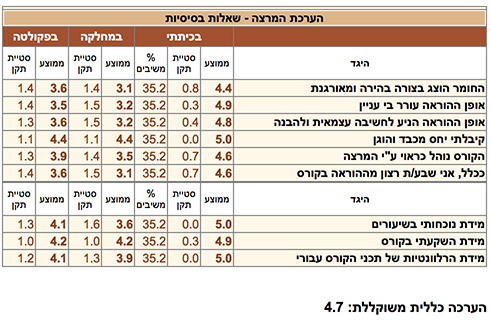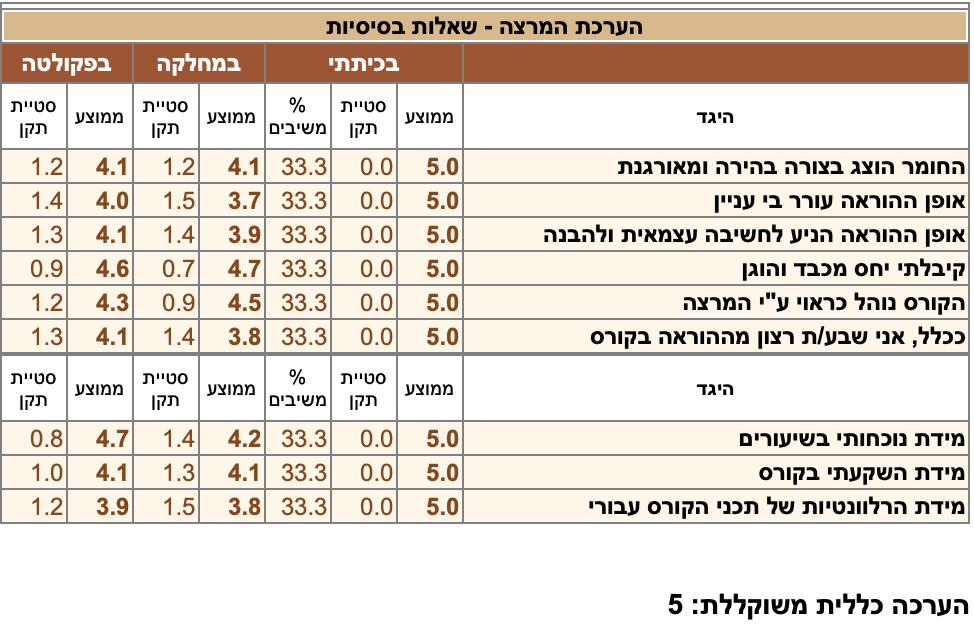Prof. Aharon Davidson - Academic Coursesa
 Course Classical Mechanics (203-1-2281) Attn. Physics (2nd year students) Books Landau & Lifshitz:  Mechanics Goldstein, Poole & Safko:  Classical Mechanics Syllabus Lagrangian formalism, Galileo principle, conservation laws, constrained systems, central field, Kepler problem, scattering, Rutherford formula, small oscillations, rigid body, Euler equations, Hamilton's equations, Poisson brackets, canonical transformations, Liouville theorem, Hamilton-Jacobi equation. Notes Teaching Survey 2018a
 Course Introduction to Particles and Fields (203-1-3421) Attn. Physics (3rd year students) Books Syllabus Poincare group, mass and spin, Tachyons, CPT, Fermions and Bosons, Lagrangian formalism, energy/momentum tensor, real/complex scalar field, global/local symmetry, U(1) gauge invariance, Maxwell equations ,Spontaneously broken symmetry, Isospin, Hypercharge, Unitary symmetry, The eightfold way, quarks and leptons,The generation puzzle, massless/massive neutrinos. Notes
a
 Course     2019 Particle Physics I (203-2-4961) Attn. Physics (graduate students) Books Syllabus The Lagrangian formalism, Self interacting scalar fields, Spontaneous breakdown of a discrete symmetry, Goldstone theorem, Higgs mechanism, Classical Non-Abelian gauge theory, The Dirac equation, the massive case, Massless Weyl (Majorana) fermions, The effective 4-Fermi theory, V-A theory, Parity non-conserevation, The minimal Glashow-Weinberg-Salam model, Massive gauge fields W and Z, The Yukawa sector, spontaneously induced fermion masses, Neutral/charged current weak interactions. Notes Teaching Survey 2019a
 Course Particle Physics II (203-2-4971) Attn. Physics (graduate students) Books Syllabus The flavor (family, generation) puzzle, Cabbibo-Kobayashi-Maskawa mixings, The GIM mechanism, K0-K0' mass difference, weak CP-violation. QCD, confinement, asymptotic freedom, Non-minimal extensions of the Glashow-Weinberg-Salam model, Strong CP-violation, the Axion model, Horizontal symmetries, Neutrino physics, the seesaw mechanism, Left-Right symmetry, Pati-salam model, B-L as the fourth color, Georgi-Glashow SU(5) minimal grand Unification, Proton decay, Neutron oscillation, SO(10), E(6) grand unifications. Notes
a
 Course    2019 Physics I (185-1-1002) Attn. Flight Academy (1st year students) Books R. Resnick, D. Halliday & K.S. Krane: Physics Vol. 2 Syllabus Kinematics, Newton's law, work and energy, linear momentum, reference frames, circular motion, harmonic motion, rigid body. Notes